Spherically Symmetric Configurations (Part II)

 |   Tiled Menu   |   Tables of Content   |  Banner Video   |  Tohline Home Page   |

Equilibrium, spherically symmetric structures are obtained by searching for time-independent solutions to the identified set of simplified governing equations. The steady-state flow field that must be adopted to satisfy both a spherically symmetric geometry and the time-independent constraint is,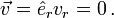$~\vec{v} = \hat{e}_r v_r = 0 \, .$

After setting the radial velocity,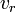$~v_r$, and all time-derivatives to zero, we see that the 1st (continuity) and 3rd (first law of thermodynamics) equations are trivially satisfied while the 2nd (Euler) and 4th give, respectively,

Hydrostatic Balance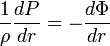$\frac{1}{\rho}\frac{dP}{dr} =- \frac{d\Phi}{dr}$ ,

and,

Poisson Equation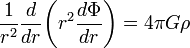$\frac{1}{r^2} \frac{d }{dr} \biggl( r^2 \frac{d \Phi}{dr} \biggr) = 4\pi G \rho$ .

(We recognize the first of these expressions as being the statement of hydrostatic balance appropriate for spherically symmetric configurations.)

We need one supplemental relation to close this set of equations because there are two equations, but three unknown functions —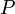$~P$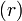$(r)~$,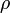$~\rho$$(r)~$, and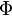$~\Phi$$(r)~$. As has been outlined in our discussion of supplemental relations for time-independent problems — and as is discussed further, below — in the context of this H_Book we will close this set of equations by specifying a structural, barotropic relationship between$~P$ and$~\rho$.

Solution Strategies

When attempting to solve the identified pair of simplified governing differential equations, it will be useful to note that, in a spherically symmetric configuration (where$~\rho$ is not a function of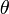$~\theta$ or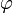$~\varphi$), the differential mass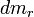$~dm_r$ that is enclosed within a spherical shell of thickness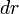$~dr$ is,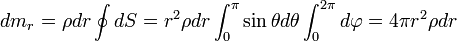$~dm_r = \rho dr \oint dS = r^2 \rho dr \int_0^\pi \sin\theta d\theta \int_0^{2\pi} d\varphi = 4\pi r^2 \rho dr$ ,

where we have pulled from the Wikipedia discussion of integration and differentiation in spherical coordinates to define the spherical surface element,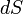$~dS$. Integrating from the center of the spherical configuration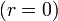$~(r=0)$ out to some finite radius,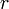$~r$, that is still inside the configuration gives the mass enclosed within that radius,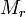$~M_r$; specifically,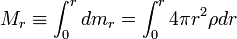$~M_r \equiv \int_0^r dm_r = \int_0^r 4\pi r^2 \rho dr$ .

We can also state that,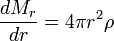$~\frac{dM_r}{dr} = 4\pi r^2 \rho$

This differential relation is often identified as a statement of mass conservation that replaces the equation of continuity for spherically symmetric, static equilibrium structures.

Technique 1

Integrating the Poisson equation once, from the center of the configuration$~(r=0)$ out to some finite radius,$~r$, that is still inside the configuration, gives,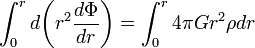$~\int_0^r d\biggl( r^2 \frac{d \Phi}{dr} \biggr) = \int_0^r 4\pi G r^2 \rho dr$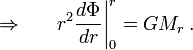$\Rightarrow ~~~~~ r^2 \frac{d \Phi}{dr} \biggr|_0^r = GM_r \, .$

Now, as long as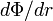$~d\Phi/dr$ increases less steeply than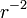$~r^{-2}$ as we move toward the center of the configuration — indeed, we will find that$~d\Phi/dr$ usually goes smoothly to zero at the center — the term on the left-hand-side of this last expression will go to zero at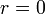$~r=0$. Hence, this first integration of the Poisson equation gives,$~\frac{d \Phi}{dr} = \frac{G M_r}{r^2} \, .$

Substituting this expression into the hydrostatic balance equation gives,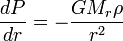$~\frac{dP}{dr} = - \frac{GM_r \rho}{r^2}$

that is, a single governing integro-differential equation which depends only on the two unknown functions,$~P$ and$~\rho$ .

Technique 2

As long as we are examining only barotropic structures, we can replace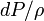$~dP/\rho$ by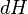$~dH$ in the hydrostatic balance relation to obtain,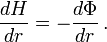$~\frac{dH}{dr} =- \frac{d\Phi}{dr} \, .$

If we multiply this expression through by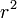$~r^2$ then differentiate it with respect to$~r$, we obtain,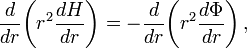$\frac{d}{dr}\biggl( r^2 \frac{dH}{dr} \biggr) =- \frac{d}{dr} \biggl( r^2 \frac{d\Phi}{dr} \biggr) \, ,$

which can be used to replace the left-hand-side of the Poisson equation and give,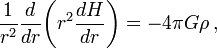$~\frac{1}{r^2} \frac{d}{dr}\biggl( r^2 \frac{dH}{dr} \biggr) =- 4\pi G \rho \, ,$

that is, a single second-order governing differential equation which depends only on the two unknown functions,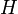$~H$ and$~\rho$.

Numerical integration examples:

Technique 3

As in Technique #2, we replace$~dP/\rho$ by$~dH$ in the hydrostatic balance relation, but this time we realize that the resulting expression can be written in the form,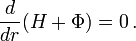$~\frac{d}{dr}(H+\Phi) = 0 \, .$

This means that, throughout our configuration, the functions$~H$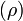$(\rho)~$ and$~\Phi$$(\rho)~$ must sum to a constant value, call it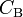$~C_\mathrm{B}$. That is to say, the statement of hydrostatic balance reduces to the algebraic expression,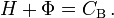$H + \Phi = C_\mathrm{B} \, .$

This relation must be solved in conjunction with the Poisson equation,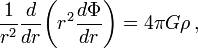$~\frac{1}{r^2} \frac{d }{dr} \biggl( r^2 \frac{d \Phi}{dr} \biggr) = 4\pi G \rho \, ,$

giving us two equations (one algebraic and the other a 2nd-order ODE) that relate the three unknown functions,$~H$,$~\rho$, and$~\Phi$ to one another.

Self-Consistent Field (SCF) Technique:

• Spherical polytropes —